# Latex公式常用符号

### 1. 公式基础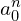—— a_{0}^{n} % 上下标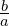—— \frac{b}{a} % 分数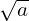—— \sqrt{a} % 根号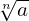—— \sqrt[n]{a} % n次方根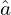—— \hat{a} % 帽子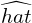—— \widehat{hat} % 大帽子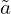—— \tilde{a} % 波浪线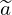—— \widetilde{a} % 宽波浪线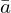—— \bar{a} % 一杆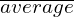—— \overline{average} % 大一杆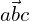—— \vec{abc} % 向量箭头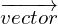—— \overrightarrow{vector} % 大向量箭头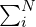—— \sum_{i}^{N} % 求和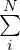—— \sum\limits_{i}^{N} % 求和（加上\limits）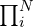—— \prod_{i}^{N} % 求乘积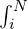—— \int_{i}^{N} % 积分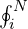—— \oint_{i}^{N} % 环路积分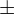—— \pm % 正负号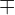—— \mp % 负正号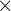—— \times % 乘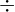—— \div % 除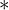—— \ast % 星星—— \cdot % 圆点—— \cdots % 三个圆点—— \vdots % 竖着三个圆点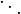—— \ddots % 斜三个圆点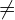—— \neq % 不等于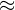—— \approx % 约等于—— \sim %波浪线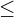—— \leq % 小于等于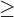—— \geq % 大于等于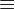—— \equiv % 恒等于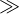—— \gg % 远大于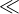—— \ll % 远小于—— \langle % 左尖括号—— \rangle % 右尖括号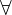—— \forall % 对于任意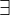—— \exists % 存在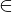—— \in % 属于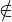—— \notin % 不属于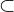—— \subset % 包含于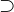—— \supset % 包含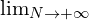——\lim_{N\to+\infty} % 极限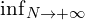—— \inf_{N\to+\infty} % 无穷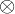—— \otimes %张量积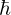—— \hbar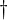—— \dag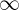—— \infty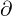—— \partial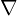—— \nabla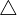—— \triangle—— \prime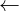—— \leftarrow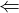—— \Leftarrow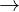—— \rightarrow（或用 \to）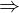—— \Rightarrow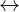—— \leftrightarrow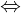—— \Leftrightarrow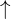—— \uparrow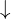—— \downarrow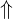—— \Uparrow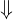—— \Downarrow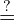—— \overset{?}{=}

### 2. 希腊字母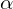—— \alpha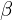—— \beta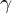—— \gamma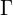—— \Gamma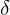—— \delta—— \Delta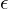—— \epsilon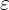—— \varepsilon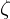—— \zeta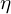—— \eta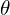—— \theta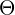—— \Theta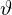—— \vartheta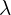—— \lambda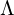—— \Lambda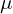—— \mu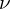—— \nu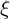—— \xi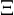—— \Xi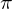—— \pi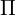—— \Pi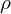—— \rho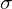—— \sigma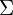—— \Sigma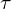—— \tau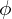—— \phi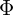—— \Phi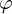—— \varphi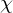—— \chi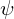—— \psi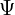—— \Psi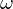—— \omega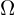—— \Omega

### 3. 换行

\begin{aligned} aligned&12345\\ &xyz\end{aligned}   % &代表对齐的位置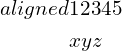### 4. 矩阵

（1）用array来写矩阵

\begin{equation*}      %开始数学环境，*代表公式不编号。或者用$\left( %左括号 \begin{array}{ccc} %该矩阵一共3列，每一列都居中放置（c代表居中​） a_{11} & a_{12} & a_{13}\\ %第一行元素，\\表示换行，&表示对齐 a_{21} & a_{22} & a_{23}\\ %第二行元素，\\表示换行，&表示对齐 \end{array} \right) %右括号 \end{equation*} 显示为（2）用matrix来写矩阵 %不带括号的矩阵 \begin{equation*} %开始数学环境，*代表公式不编号。或者用$
\begin{matrix}
a_{11} & a_{12} & a_{13}\\  %第一行元素，\\表示换行，&表示对齐
a_{21} & a_{22} & a_{23}\\  %第二行元素，\\表示换行，&表示对齐
\end{matrix}
\end{equation*}

%带()的矩阵
\begin{equation*}
\begin{pmatrix}
a_{11} & a_{12} & a_{13}\\
a_{21} & a_{22} & a_{23}\\
\end{pmatrix}
\end{equation*}

%带[]的矩阵
\begin{equation*}
\begin{bmatrix}
a_{11} & a_{12} & a_{13}\\
a_{21} & a_{22} & a_{23}\\
\end{bmatrix}
\end{equation*}

%带{}的矩阵
\begin{equation*}
\begin{Bmatrix}
a_{11} & a_{12} & a_{13}\\
a_{21} & a_{22} & a_{23}\\
\end{Bmatrix}
\end{equation*}

%行列式
\begin{equation*}
\begin{vmatrix}
a_{11} & a_{12} & a_{13}\\
a_{21} & a_{22} & a_{23}\\
\end{vmatrix}
\end{equation*}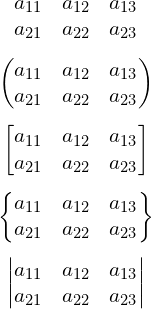### 5. 字体

（1）公式中把斜体改为正体

$\mathrm{}$

（2）黑体

$\mathbf{}$
$\bm{}$

（3）花体

$\mathbb{H}$
$\mathcal{H}$
$\mathscr{H}$

• \mathbb{}需要宏包：\usepackage{amssymb}
• \mathscr{}需要宏包：\usepackage{mathrsfs}

 LaTeX 变量用正体

 Latex中的空格

 Latex设置字体大小

8,220 次浏览

【说明：本站主要是个人的一些笔记和代码分享，内容可能会不定期修改。为了使全网显示的始终是最新版本，这里的文章未经同意请勿转载。引用请注明出处：https://www.guanjihuan.com